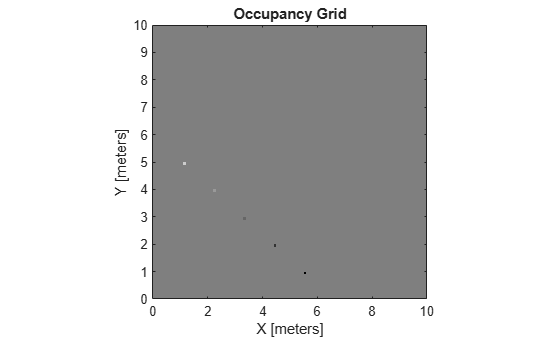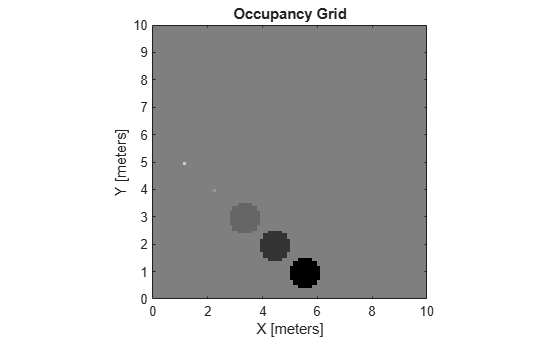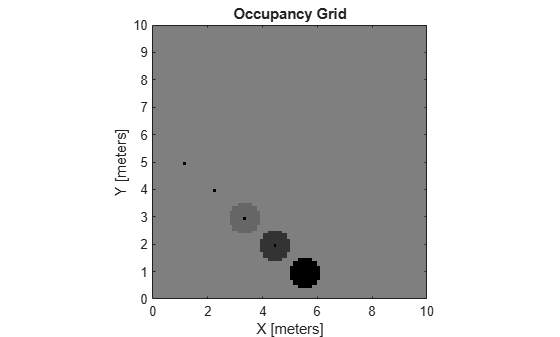world2grid

Convert world coordinates to grid indices

Description

example

ij = world2grid(map,xy) converts an array of world coordinates, xy, to an array of grid indices, ij in [row col] format.

Examples

collapse all

Create an empty map of 10-by-10 meters in size.

map = occupancyMap(10,10,10);

Update the occupancy of specific world locations with new probability values and display the map.

x = [1.2; 2.3; 3.4; 4.5; 5.6];
y = [5.0; 4.0; 3.0; 2.0; 1.0];

pvalues = [0.2; 0.4; 0.6; 0.8; 1];

updateOccupancy(map,[x y],pvalues)
figure
show(map)Inflate the occupied areas by a radius of 0.5 m. The larger occupancy values overwrite the smaller values.

inflate(map,0.5)
figure
show(map)Get the grid locations from the world locations.

ij = world2grid(map,[x y]);

Set occupancy values for the grid locations.

setOccupancy(map,ij,ones(5,1),'grid')
figure
show(map)Input Arguments

collapse all

Map representation, specified as a occupancyMap, mapLayer, or multiLayerMap object.

World coordinates, specified as an n-by-2 matrix of [x y] pairs, where n is the number of world coordinates.

Data Types: double

Output Arguments

collapse all

Grid indices, returned as an n-by-2 matrix of [i j] pairs in [row col] format, where n is the number of grid positions. The grid cell locations are counted from the top left corner of the grid.

Data Types: double